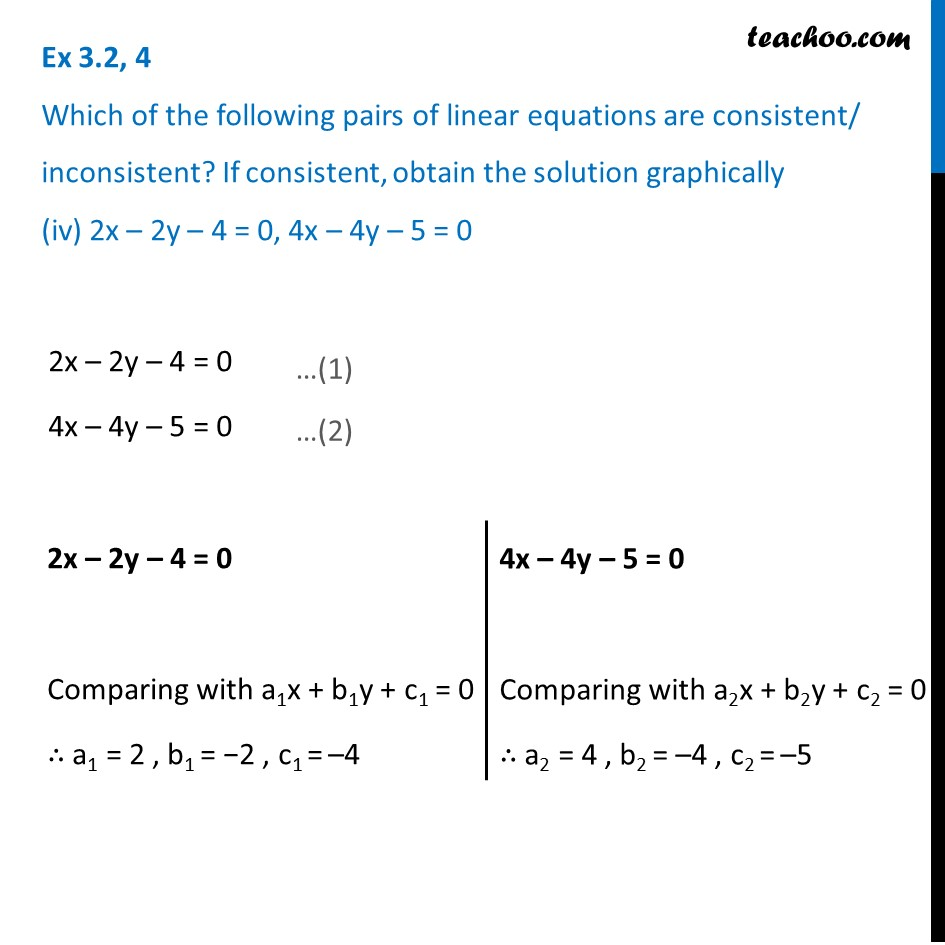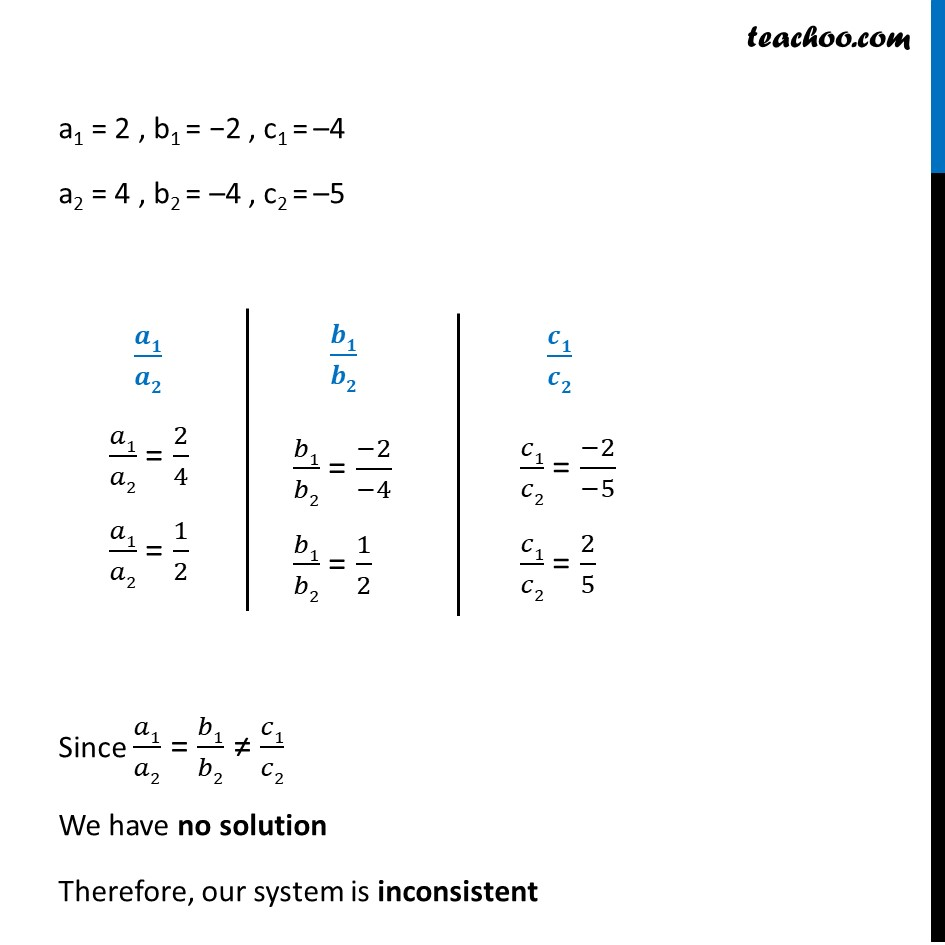1. Chapter 3 Class 10 Pair of Linear Equations in Two Variables (Term 1)
2. Serial order wise
3. Ex 3.2

Transcript

Ex 3.2, 4 Which of the following pairs of linear equations are consistent/ inconsistent? If consistent, obtain the solution graphically (iv) 2x – 2y – 4 = 0, 4x – 4y – 5 = 0 2x – 2y – 4 = 0 4x – 4y – 5 = 0 2x – 2y – 4 = 0 Comparing with a1x + b1y + c1 = 0 ∴ a1 = 2 , b1 = −2 , c1 = –4 4x – 4y – 5 = 0 Comparing with a2x + b2y + c2 = 0 ∴ a2 = 4 , b2 = –4 , c2 = –5 a1 = 2 , b1 = −2 , c1 = –4 a2 = 4 , b2 = –4 , c2 = –5 𝒂𝟏/𝒂𝟐 𝑎1/𝑎2 = 2/4 𝑎1/𝑎2 = 1/2 𝒃𝟏/𝒃𝟐 𝑏1/𝑏2 = (−2)/(−4) 𝑏1/𝑏2 = 1/2 𝒄𝟏/𝒄𝟐 𝑐1/𝑐2 = (−2)/(−5) 𝑐1/𝑐2 = 2/5 Since 𝑎1/𝑎2 = 𝑏1/𝑏2 ≠ 𝑐1/𝑐2 We have no solution Therefore, our system is inconsistent